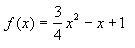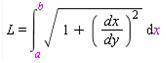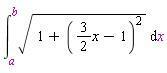# Using integral to find length of curve

• a1ccook

## Homework Statement

Ok, the problem is there are two curves and I need to find out which is shorter. I can't find the first one so I'll just post that. The other should be easy after I learn how to get this one.

## Homework Equations

The first curve is:## The Attempt at a Solution

I used the formulaand worked it down to.

I've also seen some similar problems to this where people recommended trig substitutions. I tried substituting tan u in which gave me sec^2 u under the radical. If I go that route, I don't know the integral of sec or how you could carry on. The other way I thought you could do this was to just simplify (from the last picture I posted) and try to integrate that... but that would be rough. Any tips?

The integral of sec(x) is usually done with the trick of multiplying the numerator and denominator by (sec(x) + tan(x)) followed by a u-substitution. Try it.